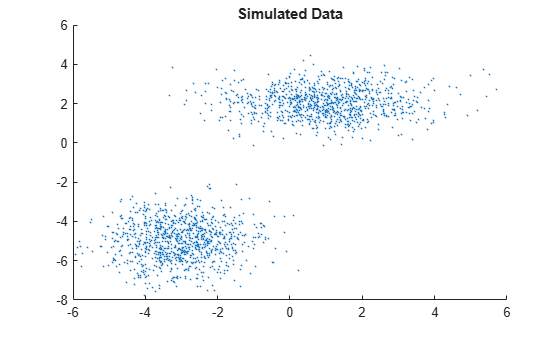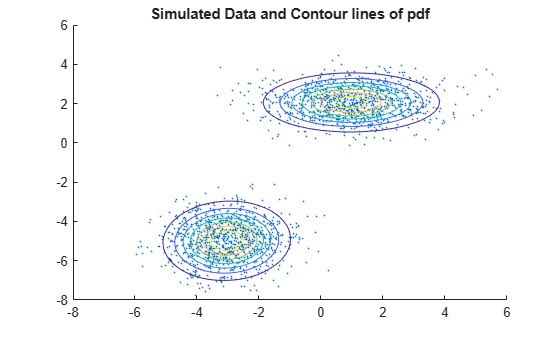# Fit Gaussian Mixture Model to Data

This example shows how to simulate data from a multivariate normal distribution, and then fit a Gaussian mixture model (GMM) to the data using `fitgmdist`. To create a known, or fully specified, GMM object, see Create Gaussian Mixture Model.

`fitgmdist` requires a matrix of data and the number of components in the GMM. To create a useful GMM, you must choose `k` carefully. Too few components fails to model the data accurately (i.e., underfitting to the data). Too many components leads to an over-fit model with singular covariance matrices.

Simulate data from a mixture of two bivariate Gaussian distributions using `mvnrnd`.

```mu1 = [1 2]; sigma1 = [2 0; 0 .5]; mu2 = [-3 -5]; sigma2 = [1 0; 0 1]; rng(1); % For reproducibility X = [mvnrnd(mu1,sigma1,1000); mvnrnd(mu2,sigma2,1000)];```

Plot the simulated data.

```scatter(X(:,1),X(:,2),10,'.') % Scatter plot with points of size 10 title('Simulated Data')```Fit a two-component GMM. Use the `'Options'` name-value pair argument to display the final output of the fitting algorithm.

```options = statset('Display','final'); gm = fitgmdist(X,2,'Options',options)```
```5 iterations, log-likelihood = -7105.71 gm = Gaussian mixture distribution with 2 components in 2 dimensions Component 1: Mixing proportion: 0.500000 Mean: -3.0377 -4.9859 Component 2: Mixing proportion: 0.500000 Mean: 0.9812 2.0563 ```

Plot the pdf of the fitted GMM.

```gmPDF = @(x,y) arrayfun(@(x0,y0) pdf(gm,[x0 y0]),x,y); hold on h = fcontour(gmPDF,[-8 6]); title('Simulated Data and Contour lines of pdf');```Display the estimates for means, covariances, and mixture proportions

`ComponentMeans = gm.mu`
```ComponentMeans = 2×2 -3.0377 -4.9859 0.9812 2.0563 ```
`ComponentCovariances = gm.Sigma`
```ComponentCovariances = ComponentCovariances(:,:,1) = 1.0132 0.0482 0.0482 0.9796 ComponentCovariances(:,:,2) = 1.9919 0.0127 0.0127 0.5533 ```
`MixtureProportions = gm.ComponentProportion `
```MixtureProportions = 1×2 0.5000 0.5000 ```

Fit four models to the data, each with an increasing number of components, and compare the Akaike Information Criterion (AIC) values.

```AIC = zeros(1,4); gm = cell(1,4); for k = 1:4 gm{k} = fitgmdist(X,k); AIC(k)= gm{k}.AIC; end```

Display the number of components that minimizes the AIC value.

```[minAIC,numComponents] = min(AIC); numComponents```
```numComponents = 2 ```

The two-component model has the smallest AIC value.

Display the two-component GMM.

`gm2 = gm{numComponents}`
```gm2 = Gaussian mixture distribution with 2 components in 2 dimensions Component 1: Mixing proportion: 0.500000 Mean: -3.0377 -4.9859 Component 2: Mixing proportion: 0.500000 Mean: 0.9812 2.0563 ```

Both the AIC and Bayesian information criteria (BIC) are likelihood-based measures of model fit that include a penalty for complexity (specifically, the number of parameters). You can use them to determine an appropriate number of components for a model when the number of components is unspecified.